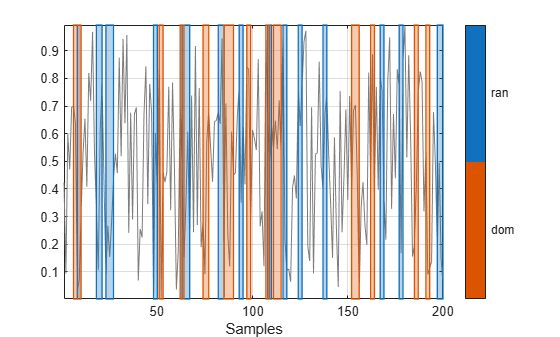# plotsigroi

Plot signal regions based on signal mask

## Syntax

``plotsigroi(msk,x)``
``plotsigroi(msk,x,patchflag)``
``h = plotsigroi(msk,x)``

## Description

example

````plotsigroi(msk,x)` plots signal `x` with color-coded regions based on the source and properties in `msk`. If `x` is complex-valued, the function plots its magnitude.```

example

````plotsigroi(msk,x,patchflag)` plots regions of interest using rectangular patches if `patchflag` is `true`.```
````h = plotsigroi(msk,x)` returns the figure handle of the color-coded plot. You can use the figure handle to query and modify figure properties.```

## Examples

collapse all

Consider a mask of binary sequences for two categories, `ran` and `dom`. Use the sequences to generate a `signalMask` object. Discard regions with fewer than 3 samples.

```rng default sq = randi(2,200,2)-1; m = signalMask(sq,"MinLength",3,"Categories",["ran" "dom"]);```

Generate a sequence of 200 random numbers. Plot the regions of interest.

```x = rand(200,1); plotsigroi(m,x)```Plot the regions of interest using rectangular patches.

`plotsigroi(m,x,true)`## Input Arguments

collapse all

Signal mask, specified as a `signalMask` object.

Example: `signalMask(table([2 4;6 7],["male" "female"]'))` specifies a signal mask with a three-sample `male` region and a two-sample `female` region.

Example: ```signalMask(categorical(["" "male" "male" "male" "" "female" "female" ""]',["male" "female"]))``` specifies a signal mask with a three-sample `male` region and a two-sample `female` region.

Example: ```signalMask([0 1 1 1 0 0 0 0;0 0 0 0 0 1 1 0]','Categories',["male" "female"])``` specifies a signal mask with a three-sample `male` region and a two-sample `female` region.

Input signal, specified as a vector.

Example: `chirp(0:1/1e3:1,25,1,50)` specifies a chirp sampled at 1 kHz.

Data Types: `single` | `double`
Complex Number Support: Yes

Rectangular patch option, specified as a logical value. `plotsigroi` uses rectangular patches to plot regions of interest if `patchflag` is `true`.

Data Types: `logical`

## Output Arguments

collapse all

Figure handle, returned as an integer scalar.

## Version History

Introduced in R2020b